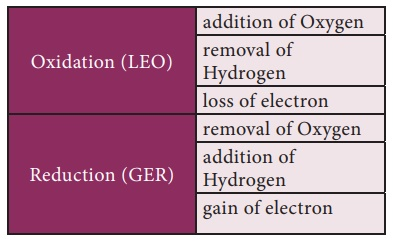Home | | Science 9th std | Oxidation, Reduction and Redox reactions

# Oxidation, Reduction and Redox reactions

Look at the following pictures. When an apple is cut and left for sometimes, its surface turns brown. Similarly, iron bolts and nuts in metallic structures get rusted. Do you know why are these happening? It is because of a reaction called oxidation.

Oxidation, Reduction and Redox reactions

Look at the following pictures. When an apple is cut and left for sometimes, its surface turns brown. Similarly, iron bolts and nuts in metallic structures get rusted. Do you know why are these happening? It is because of a reaction called oxidation.Oxidation: A chemical reaction which involves addition of oxygen or removal of hydrogen or loss of electrons is called oxidation.

2 Mg + O2 → 2 MgO (addition of oxygen)

CaH2 → Ca + H2 (removal of hydrogen)

Fe2+ →Fe3+ + e (loss of electron)

Reduction: A chemical reaction which involves addition of hydrogen or removal of oxygen or gain of electrons is called reduction.

2 Na + H2 → 2 NaH (addition of hydrogen)

CuO + H2 → Cu + H2O (removal of oxygen)

Fe3+ + e → Fe2+ (gain of electron)

Redox reactions: Generally, the oxidation and reduction occurs in the same reaction (simultaneously). If one reactant gets oxidised, the other gets reduced. Such reactions are called oxidation-reduction reactions or Redox reactions.

Ex. 1 : 2 PbO + C → 2 Pb + CO2

Ex. 2 : Zn + CuSO4 → Cu + ZnSO4## Oxidising agents and Reducing agents

Substances which have the ability to oxidise other substances are called Oxidising agents. ese are also called as electron acceptors because they remove electrons from other substances.

Example: H2O2, MnO4-, CrO3, Cr2O72-

Substances which have the ability to reduce other substances are called Reducing agents. ese are also called as electron donors because they donate electrons to other substances.

Example: NaBH4, LiAlH4 and metals like Palladium, Platinum.

## Oxidation reactions in daily life:

In nature the oxygen present in atmospheric air oxidises many things, starting from metals to living tissues.

·           The shining surface of metals tarnishes due to the formation of respective metal oxides on their surfaces. This is called corrosion.

·           The freshly cut surfaces of vegetables and fruits turns brown a er some time because of the oxidation of organic compounds present in them.

·           The oxidation reaction in food materials that were le open for a long period is responsible for spoiling of food. is is called Rancidity.

## Oxidation number

Oxidation number of an element is de ned as the formal charge which an atom of that element appears to have when electrons are counted.

Oxidation number also called Oxidation state, the total number of electrons that an atom either gains or losses in order to form a chemical bond with another atom.The sum of oxidation numbers of all the atoms in the formula for a neutral compound is ZERO. The sum of oxidation numbers of an ion is the same as the charge on that ion. Negative oxidation number in compounds of two unlike atoms is assigned to the more electronegative atom.

For example,

·           Oxidation number of K and Br in KBr molecule is +1 and -1 respectively.

·           Oxidation number of N in NH3 molecule is -3

·           Oxidation number of H is +1 (except hydrides)

·           Oxidation number of oxygen in most cases is -2

(Oxidation Number = ON)

## Problems on determination of Oxidation Number

ON of neutral molecule is always zero

### Illustration 1 – Oxidation Number of H and O in H2O

Let us take ON of H = +1 and ON of O = -2

2 X (+1) + 1 X (-2) = 0

(+2) + (-2) = 0 thus, ON of H is +1 and ON of O is -2

### Illustration 2 – Oxidation Number of Na and Cl in NaCl

ON of Na = +1 and ON of Cl = -1

(+1) + (-1) = 0 thus, ON of Na is +1 and ON of Cl is -1

### Illustration 3 – Oxidation Number of S in H2SO4

Let ON of S be (x) and we know ON of H = +1 and O = -2

2 X (+1) + (x) + 4 X (-2) = 0

(+2) + (x) + (-8) = 0

(x) = +6 therefore, ON of S is +6

### Illustration 4 – Oxidation Number of Cr in K2Cr2O7

Let ON of Cr be x and we know ON of K = +1 and O = -2

2 X (+1) + 2 X (x) + 7 X (-2) = 0

(+2) + (2x) + (-14) = 0

2x = +12

x = +6 therefore, ON of Cr in K2Cr2O7 is +6

### Illustration 5 – Oxidation Number of Fe in FeSO4

Let ON of Fe be x and we know ON of S = +6 and O = -2

42.   + 1 X (+6) + 4 X (-2) = 0

43.   + (+6) + (-8) = 0

x = +2 therefore, ON of Fe in FeSO4 is +2

## Problems:

1. Find the oxidation number of Mn in KMnO4

2. Find the oxidation number of Cr in Na2Cr2O7

3. Find the oxidation number of Cu in CuSO4

4. Find the oxidation number of Fe in FeOStudy Material, Lecturing Notes, Assignment, Reference, Wiki description explanation, brief detail
9th Science : Chemical bonding : Oxidation, Reduction and Redox reactions |